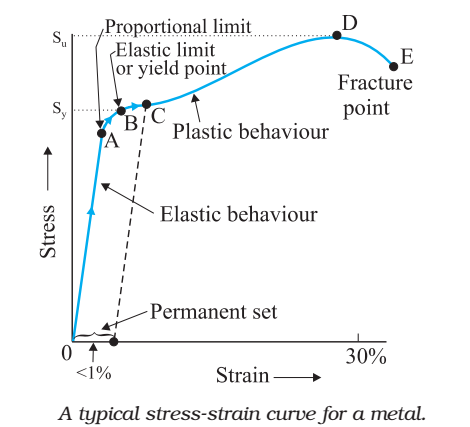Stress strain curve is used to understand the tensile strength of a given material. The given figure shows a stress-strain curve of a given metal.• The curve from 0 to A is linear. In the region, Hooke’s Proportional limit law is obeyed.
• In the region from A to 6 stress and strain are not. Proportional. Still, the body regains its original dimension, once the load is removed.
• Point B in the curve is yield point or elastic limit and the corresponding stress is known as yield strength of the material.
• The curve beyond B shows the region of plastic deformation.
• The point D on the curve shows the tensile strength of the material. Beyond this point, additional strain leads to fracture, in the given material.